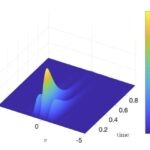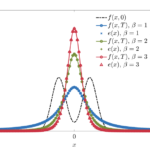# A kinetic approach to consensus-based segmentation of biomedical images

R. F. Cabini, A. Pichiecchio, A. Lascialfari, S. Figini, M. Zanella

Preprint arXiv, 2022.

In this work, we apply a kinetic version of a bounded confidence consensus model to biomedical segmentation problems. In the presented approach, time-dependent information on the microscopic state of each particle/pixel includes its space position and a feature representing a static characteristic of the system, i.e. the gray level of each pixel. From the introduced microscopic model we derive a kinetic formulation of the model. The large time behavior of the system is then computed with the aid of a surrogate Fokker-Planck approach that can be obtained in the quasi-invariant scaling. We exploit the computational efficiency of direct simulation Monte Carlo methods for the obtained Boltzmann-type description of the problem for parameter identification tasks. Based on a suitable loss function measuring the distance between the ground truth segmentation mask and the evaluated mask, we minimize the introduced segmentation metric for a relevant set of 2D gray-scale images. Applications to biomedical segmentation concentrate on different imaging research contexts.

# Micro-macro stochastic Galerkin methods for nonlinear Fokker-Plank equations with random inputsG. Dimarco, L. Pareschi, M. Zanella.

Preprint arXiv, 2022

Nonlinear Fokker-Planck equations play a major role in modeling large systems of interacting particles with a proved effectiveness in describing real world phenomena ranging from classical fields such as fluids and plasma to social and biological dynamics. Their mathematical formulation has often to face with physical forces having a significant random component or with particles living in a random environment which characterization may be deduced through experimental data and leading consequently to uncertainty-dependent equilibrium states. In this work, to address the problem of effectively solving stochastic Fokker-Planck systems, we will construct a new equilibrium preserving scheme through a micro-macro approach based on stochastic Galerkin methods. The resulting numerical method, contrarily to the direct application of a stochastic Galerkin projection in the parameter space of the unknowns of the underlying
Fokker-Planck model, leads to highly accurate description of the uncertainty dependent large time behavior. Several numerical tests in the context of collective behavior for social and life sciences are presented to assess the validity of the present methodology against standard ones.

# On a class of Fokker–Planck equations with subcritical confinementG. Toscani, M. Zanella

Atti Accad. Naz. Lincei Rend. Lincei Mat. Appl., 32:471-496, 2021. (Preprint arXiv)

We study the relaxation to equilibrium for a class linear one-dimensional Fokker–Planck equations characterized by a particular subcritical confinement potential.
An interesting feature of this class of Fokker–Planck equations is that, for any given probability density \$e(x)\$, the diffusion coefficient can be built to have \$e(x)\$ as steady state. This representation of the equilibrium density can be fruitfully used to obtain one-dimensional Wirtinger-type inequalities and to recover, for a sufficiently regular density \$e(x) \$, a polynomial rate of convergence to equilibrium.Numerical results then confirm the theoretical analysis, and allow to conjecture that convergence to equilibrium with positive rate still holds for steady states characterized by a very slow polynomial decay at infinity.